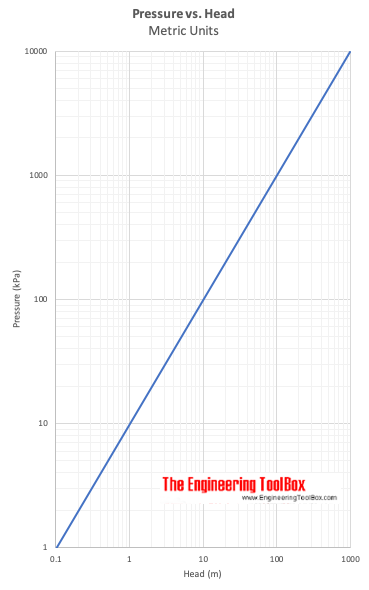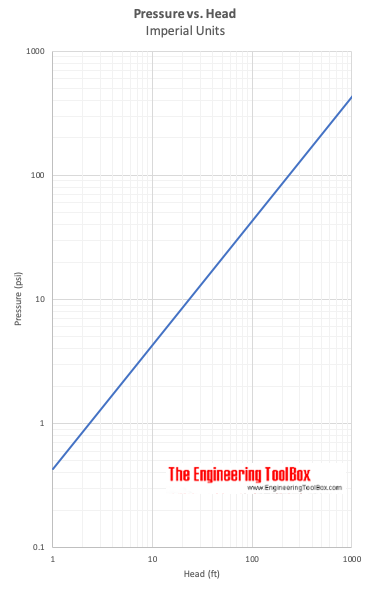Engineering ToolBox - Resources, Tools and Basic Information for Engineering and Design of Technical Applications!

## Static pressure vs. pressure head in fluids.

Pressure indicates the normal force per unit area at a given point acting on a given plane. Since there is no shearing stresses present in a fluid at rest - the pressure in a fluid is independent of direction.

For fluids - liquids or gases - at rest the pressure gradient in the vertical direction depends only on the specific weight of the fluid.

How pressure changes with elevation in a fluid can be expressed as

Δp = - γ Δh (1)

where

Δ p = change in pressure (Pa, psi)

Δ h = change in height (m, in)

γ = specific weight of fluid (N/m 3 , lb/ft 3 )

The pressure gradient in vertical direction is negative - the pressure decrease upwards.

### Specific Weight

Specific Weight of a fluid can be expressed as:

γ = ρ g (2)

where

ρ = density of fluid (kg/m 3 , slugs /ft 3 )

g = acceleration of gravity (9.81 m/s 2 , 32.174 ft/s 2 )

In general the specific weight - γ - is constant for fluids. For gases the specific weight - γ - varies with elevation (and compression).

The pressure exerted by a static fluid depends only upon

• the depth of the fluid
• the density of the fluid
• the acceleration of gravity

### Static Pressure in a Fluid

For a incompressible fluid - as a liquid - the pressure difference between two elevations can be expressed as:

Δ p = p 2 - p 1

= - γ (h 2 - h 1 ) (3)

where

p 2 = pressure at level 2  (Pa, psi)

p 1 = pressure at level 1 (Pa, psi)

h 2 = level 2    (m, ft)

h 1 = level 1 (m, ft)

(3) can be transformed to:

Δ p = p 1 - p 2

= γ (h 2 - h 1 ) (4)

or

p 1 - p 2 = γ Δ h (5)

where

Δ h = h 2 - h 1 = difference in elevation - the dept down from location h 2 to h 1 (m, ft)

or

p 1 = γ Δ h + p 2 (6)

#### Example - Pressure in a Fluid

The absolute pressure at water depth of 10 m can be calculated as:

p 1 = γ Δ h + p 2

= (1000 kg/m 3 ) (9.81 m/s 2 ) (10 m) + (101.3 kPa)

= (98100 kg/ms 2 or Pa) + (101300 Pa)

= 199400 Pa

= 199.4 kPa

where

ρ = 1000 kg/m 3

g = 9.81 m/s 2

p 2 = pressure at surface level = atmospheric pressure = 101.3 kPa

The gauge pressure can be calculated by setting p 2 = 0

p 1 = γ Δ h + p 2

= (1000 kg/m 3 ) (9.81 m/s 2 ) (10 m)

= 98100 Pa

= 98.1 kPa

(6) can be transformed to:

Δ h = (p 2 - p 1 ) / γ (7)

Δ h express head - the height difference  of a column of fluid of specific weight - γ - required to give a pressure difference Δp = p 2 - p 1 .

#### Example - Pressure vs. Head

A pressure difference of 5 psi (lb f /in 2 ) is equivalent to head in water

(5 lb f /in 2 ) (12 in/ft) (12 in/ft) / (62.4 lb/ft 3 )

= 11.6 ft of water

(5 lb f /in 2 ) (12 in/ft) (12 in/ft) / (847 lb/ft 3 )

= 0.85 ft of mercury

Specific weight of water is 62.4 (lb/ft 3 ) and specific weight of mercury is 847 (lb/ft 3 ) .## Related Topics

• ### Fluid Mechanics

The study of fluids - liquids and gases. Involving velocity, pressure, density and temperature as functions of space and time.
• ### Pumps

Piping systems and pumps - centrifugal pumps, displacement pumps - cavitation, viscosity, head and pressure, power consumption and more.

## Related Documents

• ### Darcy-Weisbach Equation - Major Pressure and Head Loss due to Friction

The Darcy-Weisbach equation can be used to calculate the major pressure and head loss due to friction in ducts, pipes or tubes.
• ### Efficiency in Pumps or Fans

The overall pump and fan efficiency is the ratio power gained by the fluid to the shaft power supplied.
• ### Hydropower

Power potential vs. head and flow rate.
• ### Hydrostatic Pressure vs. Depth

Depth and hydrostatic pressure.
• ### PE, PEH and PVC Pipes - Pressure Loss vs. Water Flow Diagram

Pressure loss (bar/100 m) and velocy in PE, PEH or PVC pipes with water flow.
• ### Potential Energy - Hydropower

Elevation and potential energy in hydropower.

Static pressure graphical presentation throughout a fluid flow system.
• ### Pressure to Head Unit Converter

Pressure vs. head units - like lb/in2, atm, inches mercury, bars, Pa and more.
• ### Pumps - Head vs. Pressure

Converting head (ft or m) to pressure (psi or bar, kg/cm2) and vice versa.
• ### Pumps - NPSH (Net Positive Suction Head)

An introduction to pumps and the Net Positive Suction Head (NPSH).

• ### Pumps - Suction Head vs. Altitude

The suction head of a water pump is affected by its operating altitude.
• ### Pumps and Fans - Energy Equation and Head Rise

The energy equation can be used to calculate the head rise in pumps and fans.
• ### System Curve and Pump Performance Curve

Utilize the system curve and the pump performance curve to select the proper pump for a particular application.
• ### Types of Fans - Capacity Ranges

Centrifugal, axial and propeller fans and their capacity ranges.

• ### Water Pressure vs. Head

Pressure in pounds per square inch (psi) vs. head in feet of water (ft h2o).

## Search

Search is the most efficient way to navigate the Engineering ToolBox.

## Engineering ToolBox - SketchUp Extension - Online 3D modeling!

Add standard and customized parametric components - like flange beams, lumbers, piping, stairs and more - to your Sketchup model with the Engineering ToolBox - SketchUp Extension - enabled for use with older versions of the amazing SketchUp Make and the newer "up to date" SketchUp Pro . Add the Engineering ToolBox extension to your SketchUp Make/Pro from the Extension Warehouse !

We don't collect information from our users. More about

## Citation

• The Engineering ToolBox (2003). Static Pressure vs. Head. [online] Available at: https://www.engineeringtoolbox.com/static-pressure-head-d_610.html [Accessed Day Month Year].

Modify the access date according your visit.

11.23.12